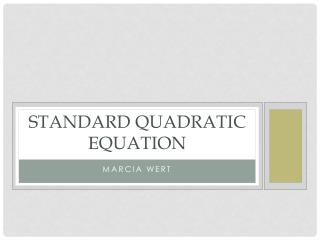# Standard Quadratic Equation - PowerPoint PPT PresentationDownload PresentationDownload Presentation- - - - - - - - - - - - - - - - - - - - - - - - - - - E N D - - - - - - - - - - - - - - - - - - - - - - - - - - -
##### Presentation Transcript

1. Standard Quadratic Equation Marcia Wert

2. STANDARD quadratic equation • Ax + bx +c = 0 2

3. STANDARD quadratic equation • 2x + 10x +12 = 0 2

4. 2 A is the number before x 2

5. b is the number before x

6. b is the number before x

7. C is WHOLE NUMBER

8. Please View the video on the trail